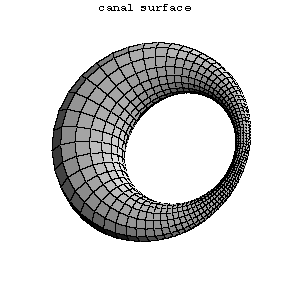# Difference between revisions of "Canal surface"

Kanalfläche

A surface for which one family of curvature lines consists of circles; the plane of each such circle intersects the surface along this circle at a constant angle. The corresponding component of the focal set of the canal surface degenerates to a curve $\Gamma$, and hence the canal surface is the envelope of a one-parameter family of spheres having the corresponding principal radii of curvature as their radii. On the contrary, if a regular curve $\zeta(s)$ is given, parametrized with arc length $s$, and $R(s)$ is the radius function for a one-parameter family of spheres with centres in $\zeta(s)$, then the canal surface enveloping this family is characterized by the following equations for its radius vector $r$: $$\langle r - \zeta(s), r - \zeta(s) \rangle = R^2(s) \,,$$ $$\langle r - \zeta(s), \zeta'(s) \rangle = -R'(s).R(s)$$ ($\langle,\rangle$ denoting the scalar product in $\mathbf{E}^3$). This equation can be solved if and only if $|R'(s)| \le 1$. If $R = \text{const.}$, then the canal surface is called a tubular surface, for which the torus may serve as an example.Figure: c020100a

A canal surface.

For the notions of a curvature line and a focal set cf. also Curvature lines, net of and Differential geometry.

Surfaces with two families of circular lines of curvature (i.e. two degenerating components of the focal set) are characterized by the cyclides of Dupin (cf. Dupin cyclide). They can be obtained as the image of the torus under a conformal transformation of the ambient space.

How to Cite This Entry:
Canal surface. Encyclopedia of Mathematics. URL: http://encyclopediaofmath.org/index.php?title=Canal_surface&oldid=18301
This article was adapted from an original article by I.Kh. Sabitov (originator), which appeared in Encyclopedia of Mathematics - ISBN 1402006098. See original article